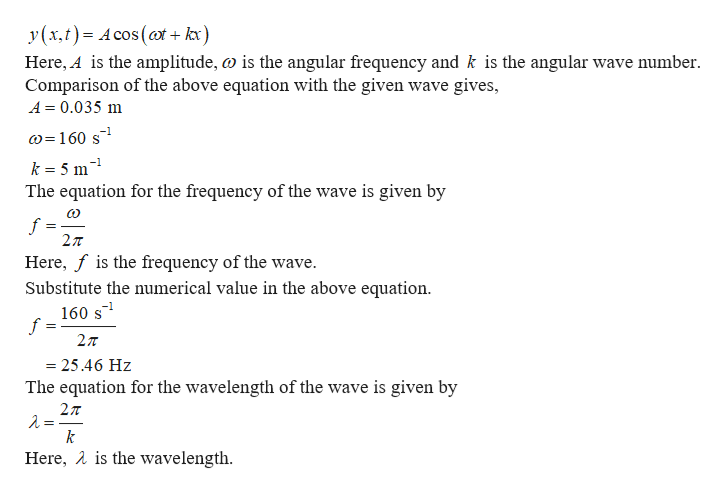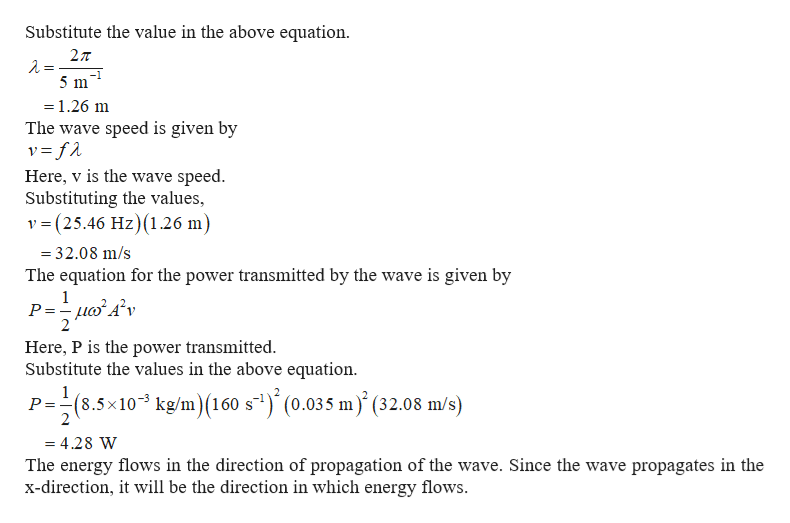# A wave of the form: y(x,t)= (0.035 m) cos[(160 s-1)t +(5 m-1) x] travels dowm a string with a density of 8.5x10-3kg/m. What is the power transmitted down the string and what is the direction of this energy flow?

Question
40 views

A wave of the form: y(x,t)= (0.035 m) cos[(160 s-1)t +(5 m-1) x] travels dowm a string with a density of 8.5x10-3kg/m. What is the power transmitted down the string and what is the direction of this energy flow?

check_circle

star
star
star
star
star
1 Rating
Step 1

The wave can be represented ashelp_outlineImage Transcriptionclosey (x, t) A cos (at+ kx) Here, A is the amplitude, o is the angular frequency and k is the angular wave number Comparison of the above equation with the given wave gives, A 0.035 m w=160 s k 5 m The equation for the frequency of the wave is given by f 27T Here, f is the frequency of the wave. Substitute the numerical value in the above equation. 160 s f 27T = 25.46 Hz The equation for the wavelength of the wave is given by 27 k Here, Ais the wavelength fullscreen
Step 2

Substitute the value in t...help_outlineImage TranscriptioncloseSubstitute the value in the above equation 27T 5 m = 1.26 m The wave speed is given by Here, v is the wave speed Substituting the values, v (25.46 Hz) (1.26 m) =32.08 m/s The equation for the power transmitted by the wave is given by 1 P =uv 2 Here, P is the power transmitted Substitute the values in the above equation 1 P =(8.5x103 kg/m)(160 s1) (0.035 m) (32.08 m/s) 2 = 4.28 W The energy flows in the direction of propagation of the wave. Since the wave propagates in the x-direction, it will be the direction in which energy flows. fullscreen

### Want to see the full answer?

See Solution

#### Want to see this answer and more?

Solutions are written by subject experts who are available 24/7. Questions are typically answered within 1 hour.*

See Solution
*Response times may vary by subject and question.
Tagged in

### Physics